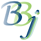# IFF() Function - Test True/False Expression

## Syntax

IFF(num_expr,true_expr,false_expr)

## Description

The IFF() function tests a specified numeric expression and returns one of two things, based on whether the expression evaluates as true (non-zero) or false (zero). Both expressions are fully evaluated, even though only one is returned, so neither expression can result in an error condition.

## Example

 READY >LET BALANCE=10 >PRINT IFF(BALANCE<0,"negative:","positive:"),BALANCE positive: 10 >LET BALANCE=-10 >PRINT IFF(BALANCE<0,"negative:","positive:"),BALANCE negative: -10 >PRINT IFF(BALANCE,balance,"zero") -10 >LET BALANCE=0 >PRINT IFF(BALANCE,balance,"zero") zero >